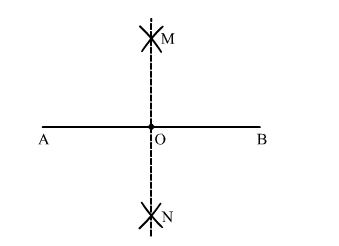# Draw a line segment AB = 5.6 cm and draw its perpendicular bisector.

Question:

Draw a line segment AB = 5.6 cm and draw its perpendicular bisector. Measure the length of each part.

Solution:Steps of Construction
1. Draw a line AB = 5.6 cm.
2. With A as centre and radius more than half of AB, draw one above and other below line AB.
3. Similarly, with B as centre draw two arcs cutting the previous drawn arcs and name the points obtained as M and N respectively.
4. Join MN intersecting AB at point O.
MN is the required perpendicular bisector.
AO = OB = 2.8 cm